# Arithmetic and Geometric Series

## Homework Statement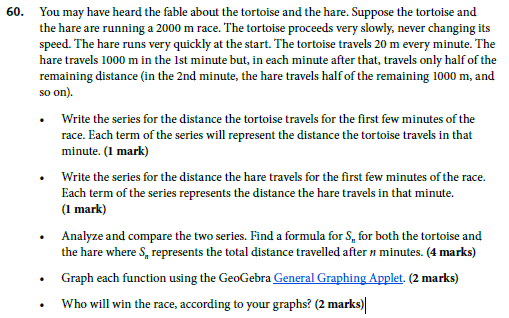## Homework Equations

no equations required

3. The Attempt at a Solution

a)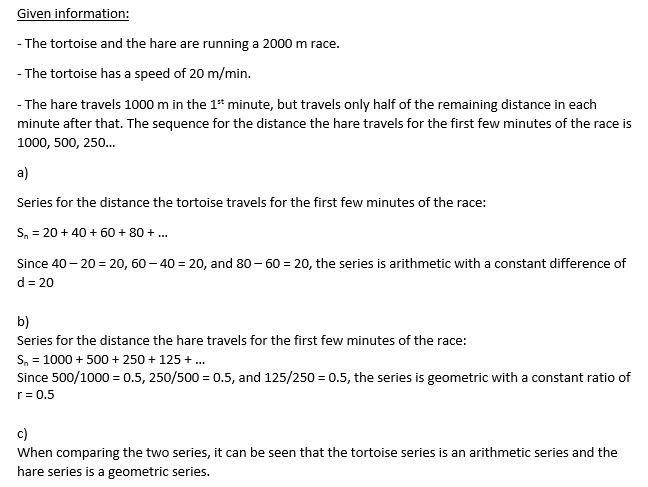so for part c) i came up with two formula's for the tortoise series:
the first formula (for the toroise series) is Sn = 20n This formula makes sense and agrees with part a). for example, if the time in minutes is 4, then the distance the tortoise travels will be Sn = 20(4) = 80 m
I came up with another formula for the tortoise series, but im not sure if this one is correct. I was following my lesson when i came up with the formula: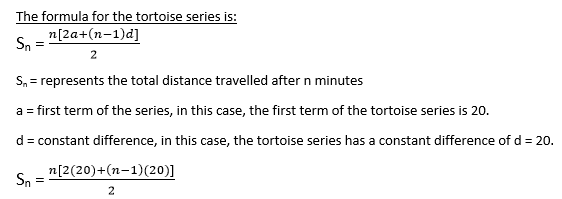I came up with a formula for the Hare series:can you please tell me which formula is the right one for the tortoise series, and also if I did the hare series formula correctly?

*For part d) of question 60, I was asked to graph each function. But im not sure what to graph, do I graph the formula's that were made in part c), or do i use the specific points from part a) and b)???

*I am aware that I am supposed to use the graphs created in part d) to answer part e). Part e) requires me to analyze the graphs and determine who wins the race, either the tortoise or hare. However, just by using the formula's, I can determine who wins. Does the following explanation make sense??

for the tortoise, the formula is Sn = 20n
I have to determine the amount of time it will take the tortoise to complete the race, so i can substitute 2000 m in replace of Sn and solve for the variable n.
2000 = 20n
2000/20 = n
100 = n
it will take exactly 100 minutes for the tortoise to complete the race.

for the hare, the formual is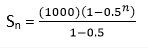i can substitue 100 minutes in replace of the variable n in the above formula for the hare, to determine the movement of the hare during the hundredth minute.
Sn = (1000)(1-0.5^100) /0.5
Sn =0.000000000000000000000000000789 meters = 0 meters

so after 100 minutes, the hare hasn't actually reached the finish line. At the hundredth minute, the tortoise passes the finish line, while the hare is moving closer to it. This means that the tortoise wins the race. I read online that in this specific problem, the hare would never actually reach the finish line (theoretically speaking).

are my answers correct?
also, please advise me on what to do for part c), d), and e)

thankyou for all the help! :D

•naoufelabs

## Answers and Replies

BvU
Science Advisor
Homework Helper
Hi,

Your 'other formula' for the tortoise series would work if it did 20, then 40, then 60 etc m in the first, second, third etc minute. That's not the case. So Sn = 20 n is the one to use.

Your S100 for the hare can't be right ! perhaps you mean something else ?

Last edited:
•late347
You did couple of things wrong.

but the most difficult part was correct (total distance travelled for hare)

a.)

tortoise part was wrong, Like this is what is being asked of you... we know that the tortoise always moves at the same speed.

If the tortoise moves at constant speed, surely that means that the tortoise moves the same distance during the 1st minute itself, as compared with the distance travelled during the 2nd minute itself. The question did not ask about the total distance. Only the distance during each individual minute.

b.) looks like this was ok

c.) looks like tortoise sum (total distance) was probably wrong. Looks like the hare sum formula (total distance) was ok.

Once again, tortoise moves at constant speed. (distance always same, per each minute time onwards).

Tortoise requires 100 minutes, in order to move 20m/1 minute. Then the tortoise finishes the race. Speed * time= distance
100min * 20meters /1min= 2000m= 2km

for the hare, the formual is View attachment 104957
i can substitue 100 minutes in replace of the variable n in the above formula for the hare, to determine the movement of the hare during the hundredth minute.
Sn = (1000)(1-0.5^100) /0.5
Sn =0.000000000000000000000000000789 meters = 0 meters

so after 100 minutes, the hare hasn't actually reached the finish line. At the hundredth minute, the tortoise passes the finish line, while the hare is moving closer to it. This means that the tortoise wins the race. I read online that in this specific problem, the hare would never actually reach the finish line (theoretically speaking).

are my answers correct?
also, please advise me on what to do for part c), d), and e)

thankyou for all the help! :D

1st one, that is incorrect,
in that formula y -value shows the total distance on the left hand side. n -value means the time taken in total. n does not show immediately how many metres were progressed during the n-th minute itself.

for the hare you decide you need to find how far the hare travels inside the time of 100 minutes.
Then you put 100 into the n, and the result gives you the total distance travelled.

Your conclusion however is correct, i think. the Tortoise does win the race because the hare "gets stuck" into the distance of 1999.999metres.
2000m seems to be the limit as n-variable approaches infinity from the left hand side..., but the hare never does reach the limit. So, the conclusion verbally is correct, I think.

ok so for part a), what I am understanding is that the tortoise series is supposed to be like this:
Sn = 20 + 20 + 20 + 20....
since you mentioned that the qts is not asking for the total distance, but the distance for each individual minute. Since the tortoise is travelling at a constant speed of 20 m/minute, this means that each minute, the tortoise is still travelling only 20 meters.
if this is the case, how would I create a formula for the tortoise series, does this series even need a formula, since all the terms are the same anyways?
also, would this series still be arithmetic, and by a constant difference of d=0 ???

so for part c), is the formula for the tortoise series supposed to be Sn = 20 n ?

Yes that sounds about right. For both tortoise issue and the tortoise total distance.

The third problem was sloppiness with regard to the hare total distance. But hare total distance was asked for. Therefore you shoud only really bother with that total distance. Not the distance, which was only mived during the 100th minute itself.

If you dont know exactly some kind of mathematical formula then it is always in my opinion better to describe it correctly verbally or in English writing. Using correct reasoning skills should give you at least partial credit.

As opposed to using wrong math formula which answers to the wrong question.

But more about the tortoise movement in the first minutes.

Tortoise moves each minute the same dstance.

Essentially the distance depends on how much time is taken.

I.e. it depends on how many terms the series has. n=number of terms=time taken
Sn= tortoise distance inthe specified time taken.

Sn= 20+20+20....
Sn= n×(20)
Repeated summation of the same number (20 metres) can be represented as multiplication here.

The A.) Question directly said that each term must be the amount of distance that the tortoise moves in that specific minute.

Therefore Sn= 20 +40+60.... is not really the true answer.

According to purplemath.com

Sequences are just some ordered line of numbers like 2,4,6,8,10... you start from 2 and add 2 always etc...

Series are summations of the terms of some sequence.

Therefore essentially series which describes the movement of the tortoise
Sn= 20×n

Thanks for the detailed explanation!
Now my only problem is with part d) and e)
I decided to graph the formula's i created in part c), and this is how it turned out.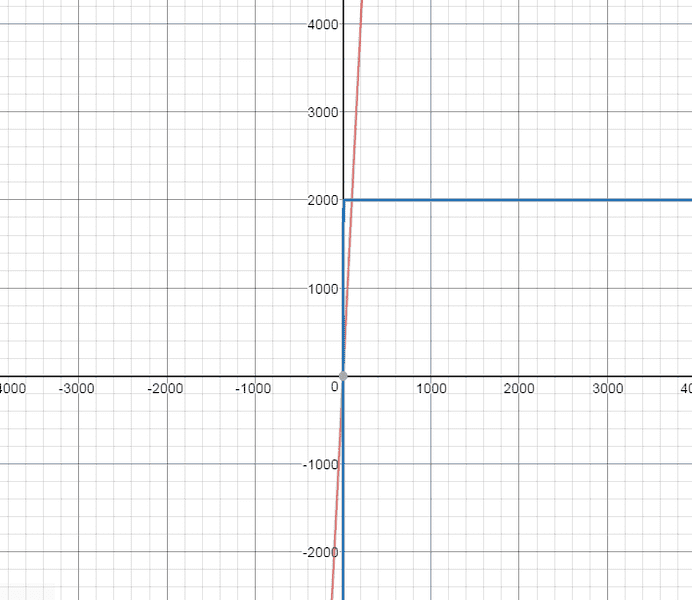the redline represents the tortoise, while the blue line represents the hare.
Using this graph, I will make an attempt to answer part e), which requires me to determine who wins the race.

In the graph above, the tortoise (represented by the red line) passes the finish line (this being 2000 m), so it is obvious that the tortoise has won the race. On the other hand, the Hare gets stuck into the distance of 1999.999 meters, indicating that the Hare never actually crosses the finish line (this explains why the graph bends at y=2000) for the Hare.

is the graph correct? and is my explanation for part e) right??
thanks for the help.

BvU
Science Advisor
Homework Helper
Graph is fine, but you want to zoom in a little on the time scale: from 0 to, say, 110 minutes instead of -4000 to + 4000....

thanks for the reply, but does the explanation of why the tortoise won make sense?